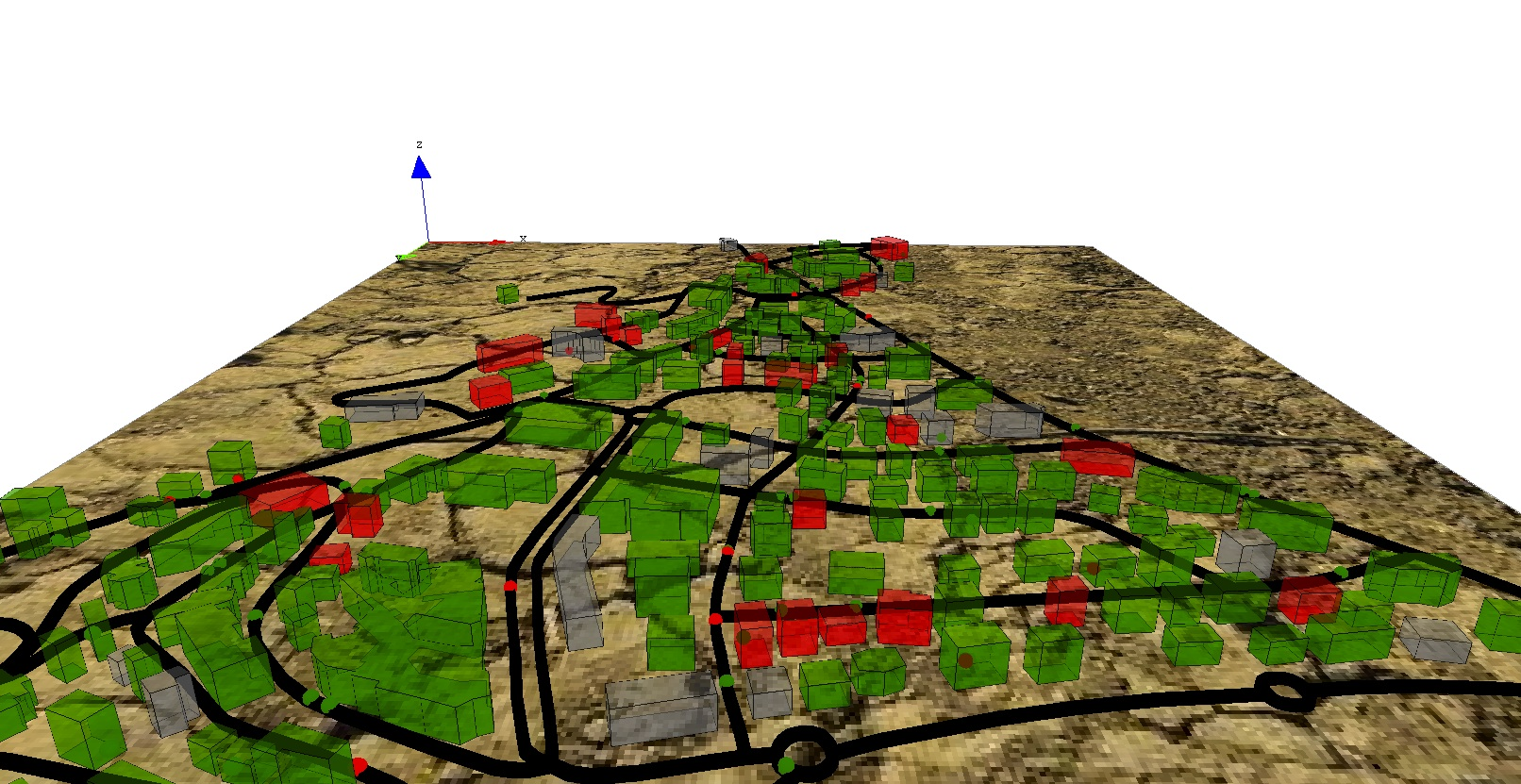Version: Next

# 7. Differential Equations

This step illustrates how to use differential equations.

## Formulation​

We are interested in the spreading of the disease inside the buildings. In order to model it, we will use differential equations. So, we will need to:

• Add two global variables to define the building epidemic properties (`beta`) and numerical integration parameter (`h`).
• Add new variables for the buildings (`I`, `S`, `T`, `t`, `I_to1`) to manage epidemic;
• Define differential equations for disease spreading inside buildings.
• Add one behavior for buildings for the spreading of the disease.## Model Definition​

### global variables​

We define two new global variables used in the disease spreading dynamic inside the buildings: (i) `beta` is the contamination rate, and `h` is the integration step (used in the `solve` statement).

``global  {    ...    float beta <- 0.01;    float h <- 0.1;    ...}``

### building​

In order to define the disease spread dynamics, we define several variables that will be used by the differential equations:

• `I`: float, number of people infected in the building.
• `S`: float, number of people not infected in the building.
• `T`: float, the total number of people in the building.
• `t`: float, the current time of the equation system integration.
• `I_to1`: float, the remaining number of people infected (float number lower between 0 and 1 according to the differential equations).
``species building {    ...    float I;    float S;    float T;    float t;       float I_to1;     ...}``

Then, we define the differential equations system that will be used for the disease spreading dynamic. Note that to define a differential equation system we use the block `equation` + name. These equations are the classic ones used by SI mathematical models.

``species building {    ....    equation SI{     diff(S,t) = (- beta * S * I / T) ;    diff(I,t) = (  beta * S * I / T) ;    }    ...}``

At last, we define a new reflex for the building called `epidemic` that will be activated only when there is someone inside the building. This reflex first computes the number of people inside the building (`T`), then the number of not infected people (`S`) and finally the number of infected ones (`I`).

If there is at least one people infected and one people not infected, the differential equations is integrated (according to the integration step value `h`) with the method Runge-Kutta 4 to compute the new value of infected people. We then sum the old value of `I_to1` with the number of people newly infected (this value is a float and not an integer). Finally, we cast this value as an integer, ask the corresponding number of not infected people to become infected, and decrement this integer value to `I\_to1`.

``species building {    ...    reflex epidemic when: nb_total > 0 {    T <- float(nb_total);    S <- float(nb_total - nb_infected);    I <- T - S;    float I0 <- I;    if (I > 0 and S > 0) {        solve SI method: #rk4 step_size: h;        I_to1 <- I_to1 + (I - I0);        int I_int <- min([int(S), int(I_to1)]);        I_to1 <- I_to1 - I_int;        ask (I_int among (people_in_building where (!each.is_infected))) {        is_infected <- true;        }    }    }    ...}``

## Complete Model​

``https://github.com/gama-platform/gama/blob/GAMA_1.8.2/msi.gama.models/models/Tutorials/Incremental%20Model/models/Incremental%20Model%207.gaml``